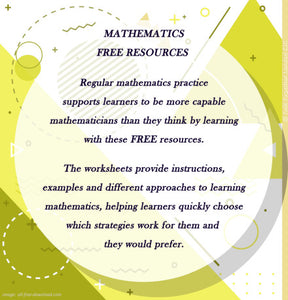after school, free, homeschool, mathematics -

# Numeracy

Some of the items listed below may not be available currently because we are updating our provision. If you have a question or need a resource urgently, please do not hesitate to contact us and we will do our best to help.

Mathematics Resources

Is it regular mathematics practice that you may be looking for?

Show learners they’re more capable mathematicians than they think by training with these worksheets!

 PRODUCT Description Addition 1 (partitioning - 2 digits for years 6 to 12) ADDITION STRATEGIES USING PLACE VALUE Addition 2 (partitioning in columns ‐ 2 digits for years 7 to 12) ADDITION STRATEGIES USING PLACE VALUE Addition 3 (partitioning ‐ 3 digits for years 8 to 12) ADDITION STRATEGIES USING PLACE VALUE Addition 4 (partitioning in columns ‐ 3 digits for years 8 to 12) ADDITION STRATEGIES USING PLACE VALUE (with zero up to 12 for years 6 to 12) ADDITION TABLE (blank with zero up to 12 for years 7 to 12) ADDITION TABLE Division 1 (partitioning ‐ TU by U for years 6 to 12) DIVISION STRATEGIES USING PLACE VALUE Division 2 (partitioning ‐ HTU by U for years 8 to 12) DIVISION STRATEGIES USING PLACE VALUE Division 3 (partitioning ‐ HTU by TU for years 9 to 12) DIVISION STRATEGIES USING PLACE VALUE (up to 12 for years 6 to 12) MULTIPLICATION SQUARE (blank up to 12 for years 8 to 12) MULTIPLICATION SQUARE Multiplication for Beginners 1 (partitioning ‐ 2 digit by 1 digit for years 6 to 12) MULTIPLICATION STRATEGIES USING PLACE VALUE Multiplication 2 (partitioning expanded column ‐ 2 digit by 1 digit for years 7 to 12) MULTIPLICATION STRATEGIES USING PLACE VALUE Multiplication 3 (partitioning ‐ 2 digit by 2 digit for years 8 to 12) MULTIPLICATION STRATEGIES USING PLACE VALUE Multiplication 4 (partitioning expanded column ‐ 2 digit by 2 digit for years 8 to 12) MULTIPLICATION STRATEGIES USING PLACE VALUE Subtraction 1 (partitioning in columns without decomposition ‐ 2 digits for years 6 to 12) SUBTRACTION STRATEGIES USING PLACE VALUE Subtraction 2 (partitioning in columns without decomposition ‐ 3 digits for years 7 to 12) SUBTRACTION STRATEGIES USING PLACE VALUE Subtraction 3 (partitioning in columns with decomposition ‐ 2 digits for years 7 to 12) SUBTRACTION STRATEGIES USING PLACE VALUE Subtraction 4 (partitioning in columns with decomposition ‐ 3 digits for years 8 to 12) SUBTRACTION STRATEGIES USING PLACE VALUE

The worksheets provide instructions, examples and different approaches to learning mathematics, helping learners quickly figure out which strategies work for them.

Skills and Topics:
Counting, addition, subtraction, multiplication, division, patterns, rounding off, negative numbers, prime numbers, factors, multiples, fractions, decimals, percentages, time, units and measures, geometry, reasoning and general problem-solving and more.

#### FREE RESOURCES

Note: Both PDF documents contain forms. You may download, print and complete or learners may complete the exercises on a computer.

There is a FREE version of the 7-sentence story template available online here:

https://talktogetherlondon.org/blogs/resources-and-services/story-maker

#### BLOG

Together: News n'Info

A chance to catch up with news, gain insight on issues and share views.